Start typing, then use the up and down arrows to select an option from the list.## General Chemistry

Learn the toughest concepts covered in Chemistry with step-by-step video tutorials and practice problems by world-class tutors

17. Acid and Base Equilibrium

# Lewis Acid and Base

In the 1920s, Gilbert Lewis proposed a new set of definitions for acids and bases.

Understanding Lewis Acids and Bases

According to the Lewis definition an acid is an electron pair acceptor.

1
concept

## Understanding Lewis Acids4m
Play a video:
Hey, guys, In this new video, we're gonna take a look at the last and final type of acids and bases the Lewis acid and the Louis space. So let's take a look. We're going to stay. In the 19 twenties, we had Gilbert Lewis. He proposed his own new definition of acids and bases. He basically did this almost the same exact time as Bronston and Laurie. Now here he defined a Lewis acid as something that accept electrons. So we're gonna say Lewis Lewis acid is an electron except er and to understand the Lewis acid, we have to basically figure out what are the different types of Lewis acids now? The first one say, We say, If we have a church acts as a lewis acid when connected to a very electoral negative element, the electro negative elements are oxygen, nitrogen, sulfur, phosphorus or the halogen zones groups seven A. So I'm talking about flooring, chlorine, bromine or iodine. Now think about it here ages. Let's think of being connected to some of these elements. Since all of these elements are more electoral negative, they're gonna be partially negative. And since H is less electoral, negative is gonna be partially positive. We tend to think of this type of Lewis acid when we have a choice. Single bonded to one of these electro negative elements. Okay, so that's the only time we really look at it. And we're talking about the bond between H and one of these electoral negative elements and think about it. Electrons are negatively charged, since their negatively charged, they'll be attracted to something that's positive or, in this case, partially positive. That's what that Delta positive means. It means it's partially positive, a little bit positive. So it's in this case when we're gonna say H can act as a Lewis acid. Now. The second type is anytime we have a positively fully positively charged hydrogen or medals. So here, H positive would be an example of a Lewis acid because it accepts electrons, electrons or negative. It's positive opposites attract. If we have positive medals, since they're all positive, they'll attract negative electrons. And then the last and final type of lewis acid is any time you're central element has less than eight valence electrons around it. Remember, this is the octet role are central Element wants to have eight electrons so they could have a similar configuration as the noble gasses. But we're gonna say Group two Way and three elements and transition metals, when they're in the center, tend to have less than eight valence electrons. For example, we could have boron. It's in Group three, so it makes three bonds. And remember, for every bond we make, we're sharing those electrons. So here boron has six electrons around it. It still has room to accept two more. That's why boron try floor. I can act as a lewis acid because the central element can accept more electrons. We could also have B E connected to two seals here. B has four electrons around it so could accept more electrons. Another electron pair. To get closer to the octet rule. We could have aluminum try bromide or aluminum, bro might actually here has six around it is well, and then a transition Metal Common Ones Inc here it has four electrons around it as well. Okay, so this last type of Lewis acid is common with groups to weigh three A and the transition metals. When they're in the center, they tend to have less than eight electrons, and as a result they serve as Lewis acids. They're gonna accept an electron pair

When hydrogen is connected to an electronegative element such as P, O, N, S or halogens then it gains a partially positive charge, which makes hydrogen act as a Lewis acid.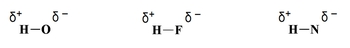Since these metal ions accept negative electron pairs then some Lewis Acids may be positively charged.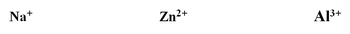If the central element has less than 8 valence electrons around it then it is more likely to accept an electron pair to follow the octet rule.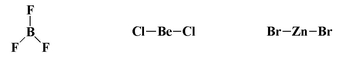According to the Lewis definition a base is an electron pair donor.

2
concept

## Understanding Lewis Bases1m
Play a video:
Now, if the Lewis acid is an electron pair except her, that would have to mean that the Lewis base is an electron pair donor. Now, what makes Luis Spaces? We're gonna say Lewis spaces are compounds with lone pairs. See here all of these what they have in common is they all have lone pairs. So they're all those spaces. And then we're gonna say, also compounds with what do the all of these have in common? They all have in common a negative charge. Okay, if you have a negative charge, that means you have an excess of extra electrons, so excess of extra lone pairs. So remember, if you're an electron paired donor, you have to have a lone pairs and or a negative charge. Negative charge just means you have extra electrons hanging around. We may not see them, but we know they're there. So this definition is a little bit different than the others. And honestly, I think it's the most difficult out of the three. Because when you get to organic chemistry, organic chemistry is predominantly the chemistry of Lewis acid and base is. That's why this type of theory seems a little bit different from the others because it it resides. Maurin, the organic chemistry region okay than general chemistry. But you still need to know it. So again, remember, Lewis acid is an electron pair, except, er ah Lewis base is an electron pair donor.

Compounds that have lone pair(s) on a central element can act as Lewis bases.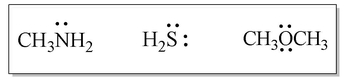Compounds that possess a negative charge can act as Lewis Bases.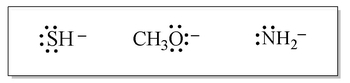3
example

## Identify each of the compounds in the following chemical equation2m
Play a video:
So here it says, identify each of the compounds in the following chemical equation. Now remember, we're dealing with Lewis acids and bases here. Ah, Lewis Acid is an electron pair, except er and Louis bases, an electron pair donor. We can clearly see from the question and from the illustration that this oxygen here, which has lone pairs on it, it looks like it's getting over that lone pair to the aluminum here. Remember, aluminum is in Group three A. We said that Group Three elements. They tend to have less than eight electrons around them in their lewis dot structures. Therefore, they can accept another lone pair to get closer to the octet role. So the oxygen here, which is donating its lone pair, is acting as a Louis base. The aluminum is accepting the lone pair, so it's acting like a Lewis acid here realize that in this process we're adding them together and because you're adding them together, that's why the product for Lewis acid based reaction is called an add duct. Now also realize a couple things are happening here. Oxygen, which had sole possession of this lone pair, is now sharing it with the aluminum. Therefore, it's gonna have a formal charge of plus one. And then the aluminum is basically gaining some new electrons from the oxygen because it's gaining new electrons it didn't have before. Its formal charge now is minus one. Here already gave us the formal charge within the product, but always be on the lookout for this. If electrons are being shared that weren't previously being shared, this is going to cause a change in our formal charges. So always be on the lookout for that whose formal charges would change. Those that tend to share electrons that they had full possession off will become more positive. Those that gain electrons they didn't have before will become more negative. Remember a nascent and a base under the Louis definition. They add together. And that's why the product it's called an add duct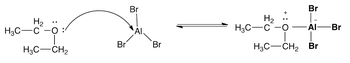4
Problem

Identify the Lewis acids and bases in the following reactions.

a) H+ + OH ⇌ H2O

b) Cl + BCl3 ⇋ BCl4

c) SO3 + H2O ⇌ H2SO4

5
Problem

Identify each of the following compounds as either a Lewis acid, a Lewis base or neither.

a)  ZnCl2                                                                                                           b)  CN

c)  NH4+                                                                                                           d) Co3+